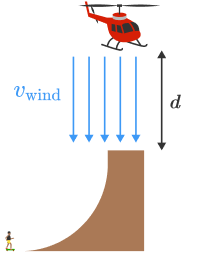# Death defying skateboard stall

Skateboarder Bob Burnquist has big ramps in his backyard that he's always trying to do bigger and better things with. Recently, Bob had a helicopter pilot hover over his quarterpipe so that he could air and stall on the helicopter's skids.Due to gravity alone Bob has to go pretty fast to air out of the quarterpipe at all but the helicopter provides a massive downward thrust that requires Bob to go even faster. This is a terrifying experience because as you approach the ramp you feel like you have enough speed to air through the blades.

The crucial factor that prevents this is that the air being pushed through the blades works to decelerate Bob's ascent. We can model the drag force on Bob as $\mathbf{F}_\textrm{d} = \frac12 \rho c_\textrm{d} A v_\textrm{relative}^2,$ where $v_\textrm{relative} = v_\textrm{wind} + v_\textrm{Bob}.$

Find Bob's speed at the top of the ramp (in $\si[per-mode=symbol]{\meter\per\second}$) such that he comes to rest exactly at the height of the skids, $d=\SI{4}{\meter}$ above the ramp.

You may want to use the code environment below.

Assume: $v_\textrm{wind} = \SI[per-mode=symbol]{30}{\meter\per\second}$ is the steady windspeed of the air coming down through the blades, Bob's cross sectional area is $A = \SI{1.32}{\meter\squared},$ the drag coefficient for the human body is $c_\textrm{d} = 1,$ $\rho=\SI[per-mode=symbol]{1.22}{\kilo\gram\per\meter\cubed},$ $g=\SI[per-mode=symbol]{10}{\meter\per\second\squared},$ and $m_\textrm{Bob} = \SI{90}{\kilo\gram}.$

import matplotlib.pyplot as plt

g = 10
v_wind = 30
m_bob = 90
C = 0.5 * 1.22 * 1.32

time = 0
height = 0
velocity = 1 # <- You'll want to experiment with this initial value.

(times, heights) = ([], []) # <- Arrays to collect the values of height and time.

# Write code to propagate height and velocity forward in time and
# collect them in the lists, times and heights.

# Uncomment this code to plot h as a function of time.
# plt.plot(times, heights)
# plt.title('$h_\mathrm{max}$ is %f m' % heights[-1])
# plt.xlabel('Time ($t$)')
# plt.ylabel('$h_\mathrm{max}$')
# plt.savefig("my_plot.png")
Python 3
You need to be connected to run code

×